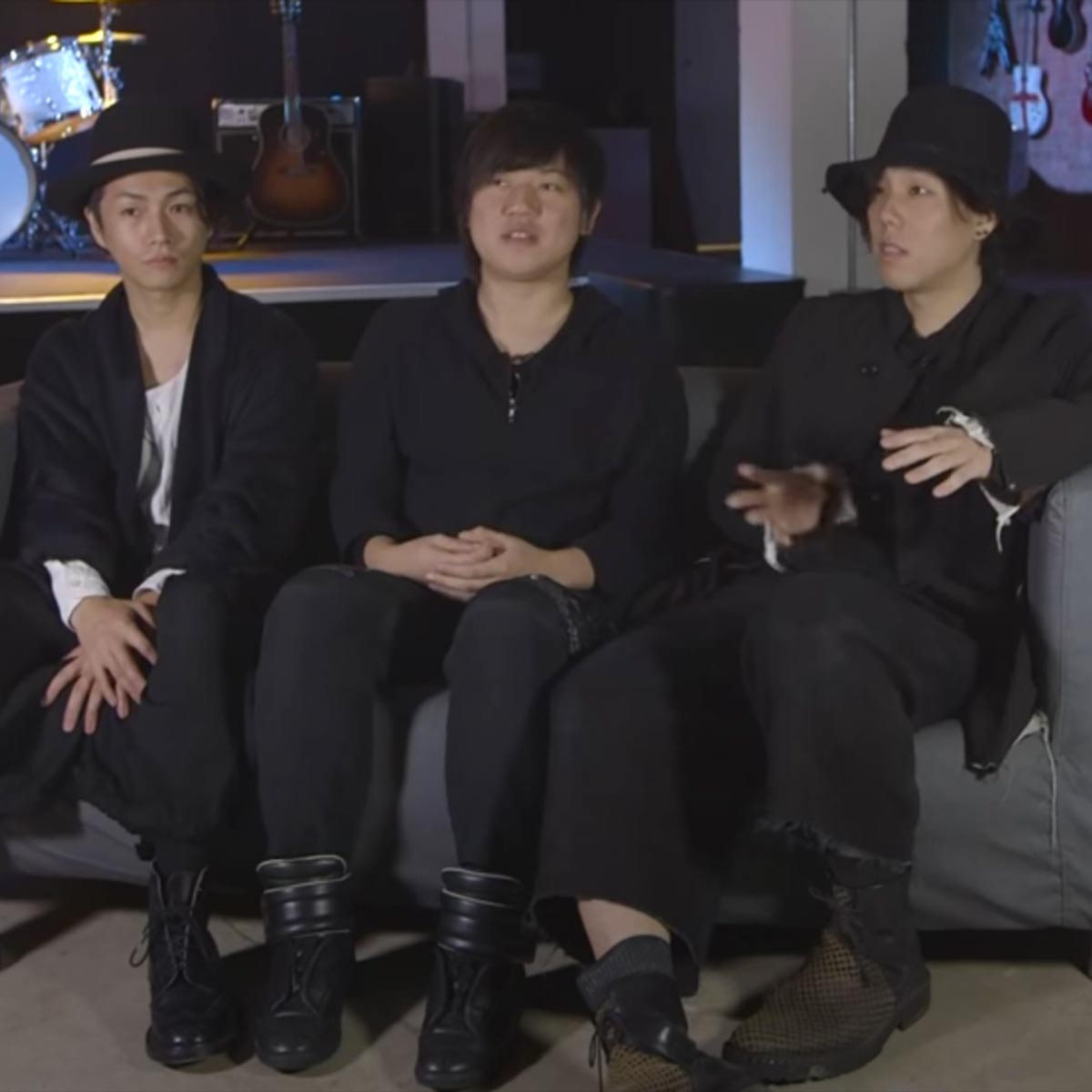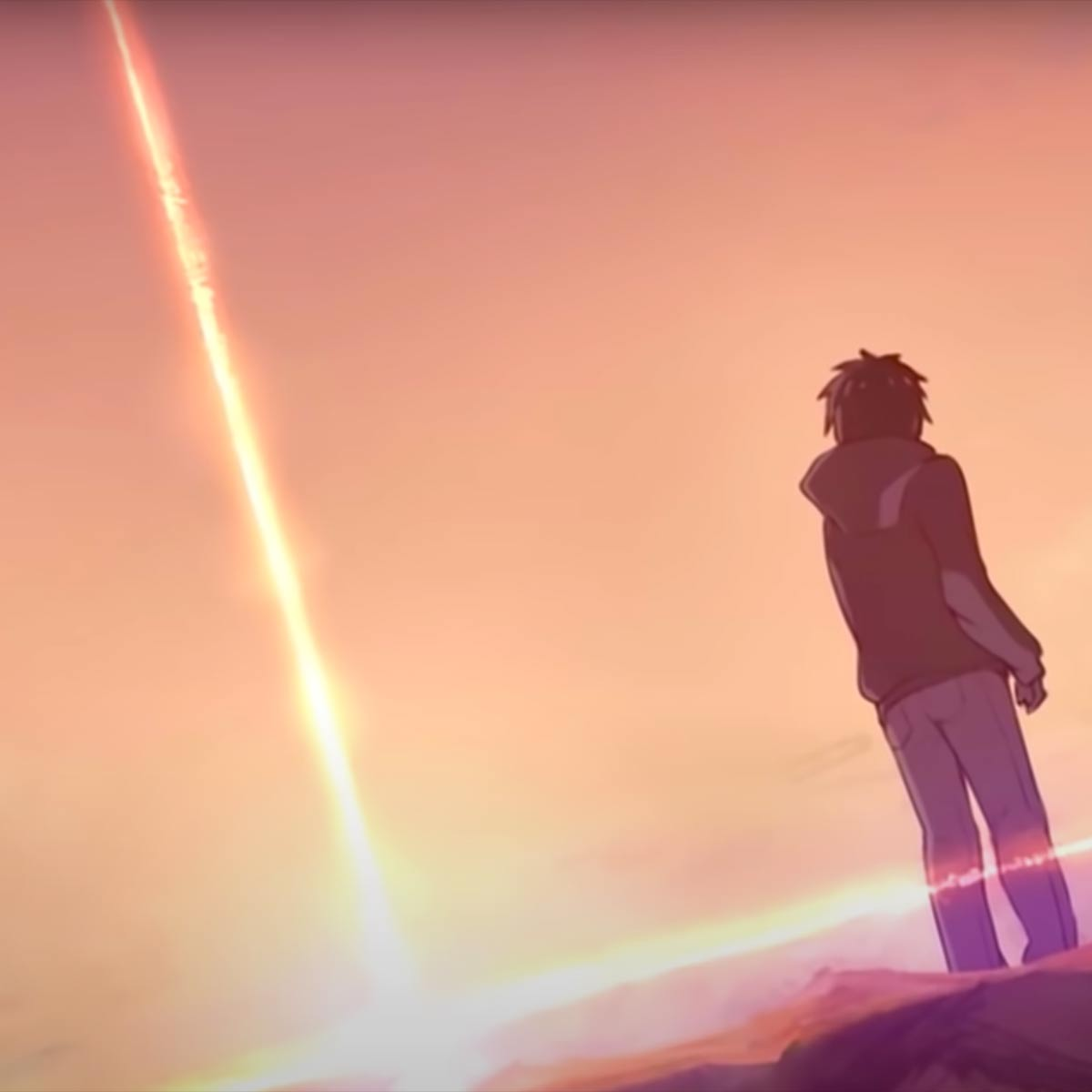3 Music Sheets

• ###### Genres:

Radwimps (stylized as RADWIMPS) is a Japanese rock band, who debuted independently in 2003 and later on major label Toshiba EMI in 2005. The band's name, Radwimps, was formed from two English language slang terms, "rad" and "wimp". According to the band, the coined word had several meanings, including "excellent weakling" and "superlative coward." The band achieved great commercial success in 2006 with their album Radwimps 4: Okazu no Gohan and are best known for their later singles "Order Made" (2008) and "Dada" (2011), both of which hit number one on Oricon's single charts; as well as for providing the soundtrack to Your Name, the highest grossing Japanese animated film.

## Artist's Music Sheets

•s d [qf] h [wg] f [0d] s [qf] o p [qs] d [wf] a [0s] o [qp] s d [qf] j [wh] f [0d] s [qf] o p [qs] d [wf] a [0s] o [qp] [qs] h s h s [hl] s h s h s [es] h s h s [hl] w s d s f s [qs] h s h s [hl] s h s h s [es] h s h s [hl] w l k h j f h a [qs] h s h s p s h j s h s h s [es] h s h s b x w s d s f z x [qs] d s f s s d s f s [qs] d s f s j d h [qs] h s f s s d s f s [qs] h s f s s d [if] h [og] f [ud] s [if] o p [is] d [of] a [us] o [ip] s d [ifh] j [ohl] f [uhx] f [ifl] o p [iops] d [of] a [us] o [ipd] s d [qf] h [wg] f [0d] s [qf] o p [qs] d [wf] a [0s] o [qp] s d [qf] h [wg] f [0d] s [qf] o p [qs] d [wf] a [0s] o [qp]
Level: 7
Intermediate
##### Mitsuha Theme (Kimi no Na wa)

•[6uh] || [qid] || [tif]o || w t || [6ih] ||| [9qysg^P] || g ||| s |||||||| [ph] h h[ih] f d ds[sif] ||| o s [ph] h s[il] kjh hj[sh] fgf[od] || s [ph] h h[ih] f d ds[tf] ||| w o sdfdessps ssq i sdfdtos h sdw o sdfdessps ssq i sdfdt o h sdw || sdse || [dfhE] ||| [tf] ||| [hl] ||| [es] || [Esd] ||| [tf] |||||| [esh] p h h[qh] f [isdf] ds[tf] ||| w [af] s [eh] h s[ql] kj[iosh] hj[th] ofgf[wd] [af] s [eh] h h[qh] f [isdf] ds[tf] ||| w o[asf]dfdessps ssq i [os]dfdtos h sdw o [sf]dfdessps ssq i [sf]dfdtos h sd[wa] o tyuy[6sf]ttet tt4 q tyuy8wt o ty[5h] [wod] tyuy6ttet tt4 q tyuy[8s]wt owty5 w tyuy[6l] [tk] [th] [ej] [td] h [tf] [tj] [4d] h [qf] j [tdf] [yj] [uh] [yf] [8l] [wk] [th] j [od] [wfk] [th] [yj] [5d] [fk] [wh] [ts] y u y [6l] [tk] [th] [ejv] [td] h [tfx] [tj] [4d] h [qf] j[td] [yh] [uf] [yj] [8lz] [wk] [th] hok[tl] [uj] [5f] k [wl] h [tf] [yj] [ul] [yj] [6lx] [tk] [th] [ejz] [td] [hv] [tfx] [tj] [4d] h [qf] j [td] [yh] [uj] [yj] [8l] [wk] [th] [hj] [od] [fh] [thl] [yj] [5d]f [wh]j [toh][yoh][toh][60us] || [^qo] ||| [8u] |||||| [60et] || [^Eqy] |||| [8wtu]
Level: 8
Expert
##### Kataware Doki (Kimi no na wa)

•s d [qf] h [wg] f [0d] s [qf]. o p [qs] d [wf] a [0s] o [qp] s d [qf] j [wh] f [0d] s [qf] o p [qs] d [wf] a [0s] o [qp] [qs] h s h s [hl] s h s h s [es] h s h s [hl] w s d s f s [qs] h s h s [hl] s h s h s [es] h s h s [hl] w l k h j f h a [qs] h s h s p s h j s h s h s [es] h s h s b x w s d s f z x [qs] d s f s s d s f s [qs] d s f s j d h [qs] h s f s s d s f s [qs] h s f s s d [if] h [og] f [ud] s [if] o p [is] d [of] a [us] o [ip] s d [ifh] j [ohl] f [uhx] f [ifl] o p [iops] d [of] a [us] o [ipd] t y [qu] o [wi] u [0y] t [qu] w e [qt] y [wu] r [0t] w [qe] t y [qu] o [wi] u [0y] t [qu] w e [qt] y [wu] r [0t] w [qe]
Level: 5
Intermediate